LSU
Mathematics

# Results

##### Input DataFig 1. Perfect & Delta x and & Delta y values for a diagonal line.
##### Figure 1 displays generated (ideal) x (left) and y (right) inputs for a diagonal line. Figure 2 displays true data from the puck for a (different) diagonal line. While true input data are noisy, preliminary attempts at noise reduction with median filtering did not improve path reconstructions, suggesting that noise is not contributing significantly to reconstruction inaccuracies. However it may be worthwhile to try more sophisticated smoothing techniques to improve the program.Fig 2. Observed & Delta x and & Delta y values for a diagonal line.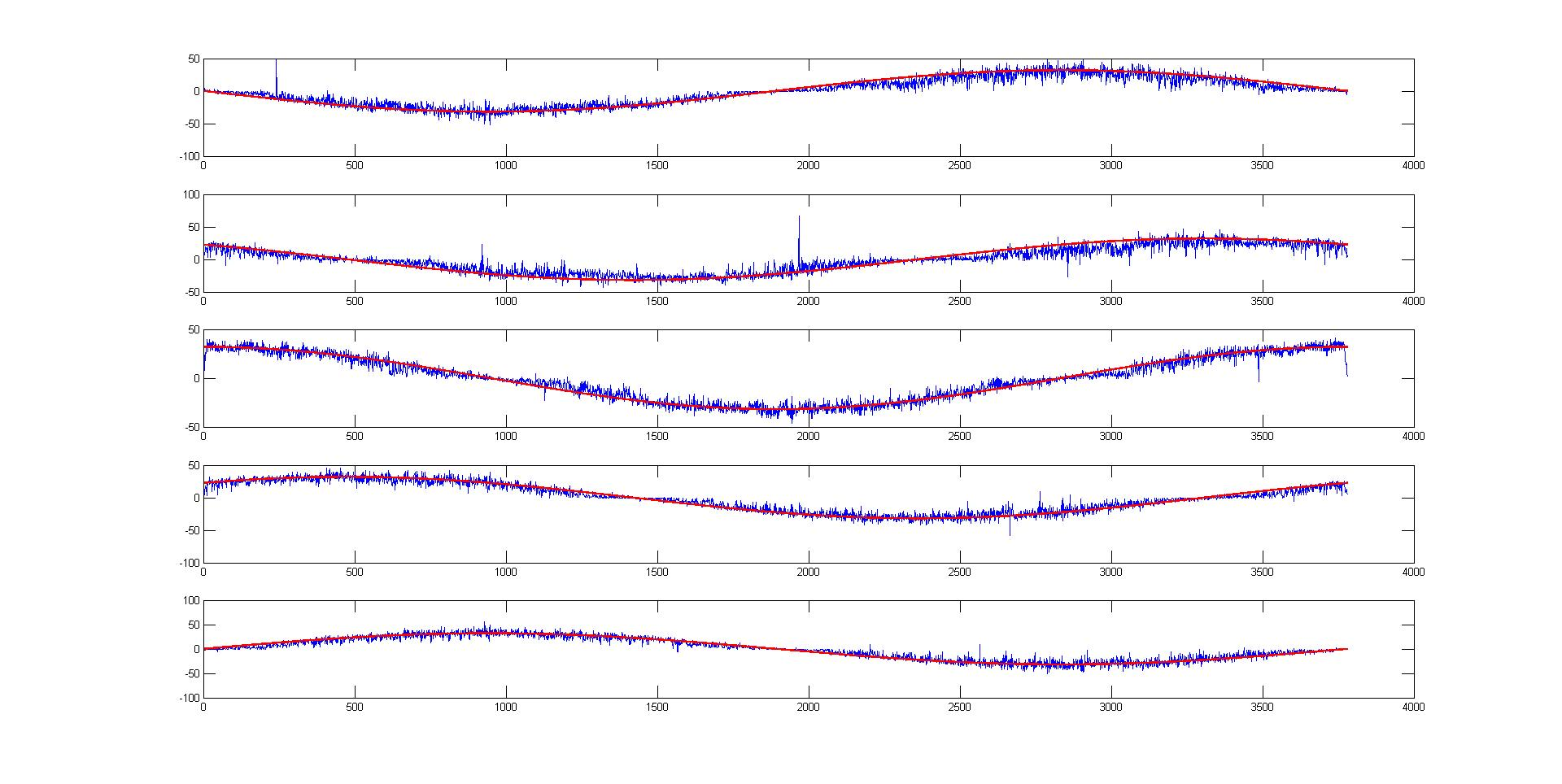Fig 3. Perfect (red) and observed (blue) Delta x values for a circle without rotation, sensors 3, 4, 5, 6, and 7.

## Path Reconstructions

##### Below are examples of paths reconstructed using our program. In the core algorithm, at each time step the change in the puck's position is computed separately using each pair of sensors, and then averaged over to produce the "true" change in position. There are also options to include the calibration and/or consistency check when reconstructing the path.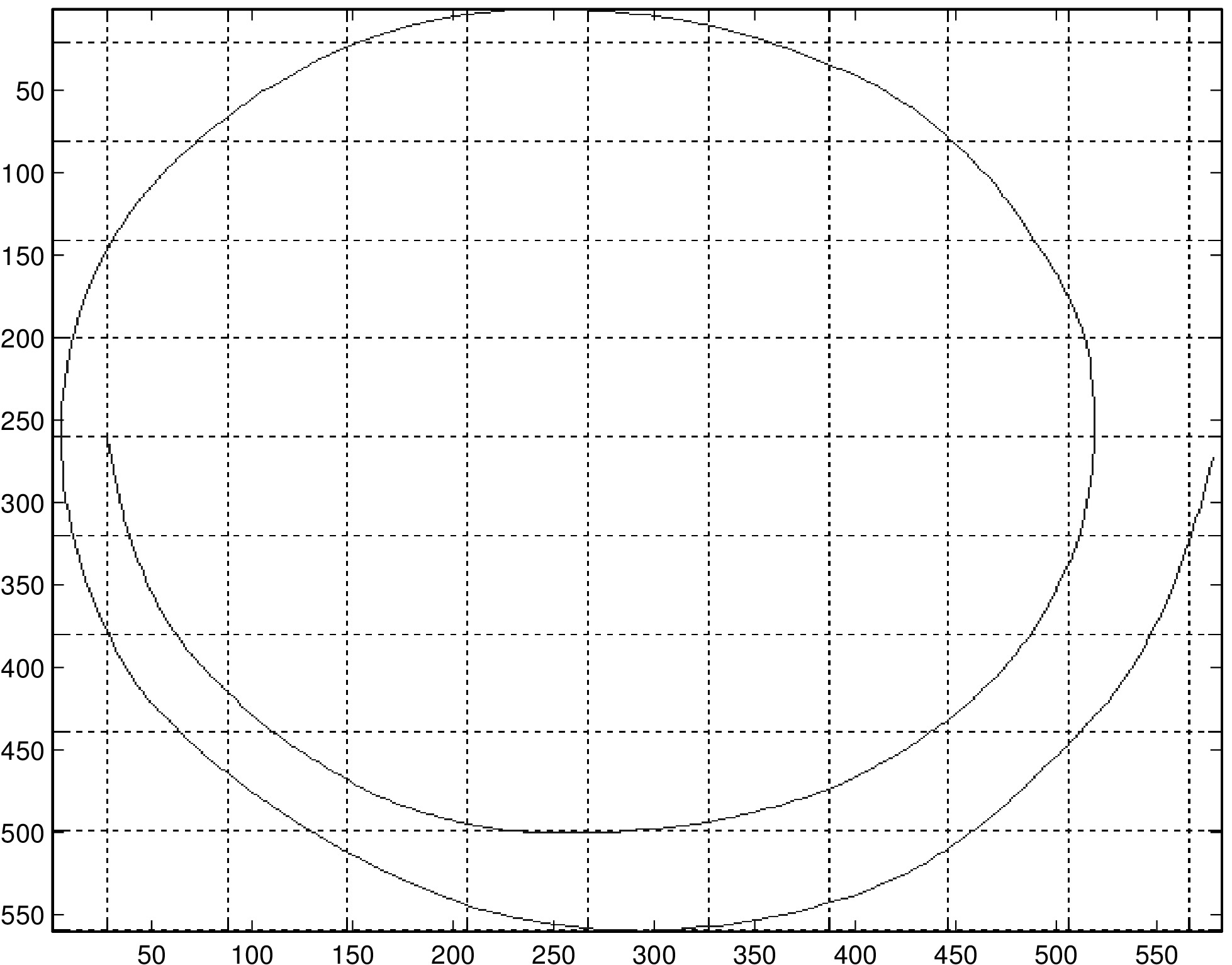Fig 4. Reconstruction of a diagonal line before (left) and after (right) consistency check.
##### Here we reconstructed the path of the same diagonal line with and without the consistency check. In this trial, three of the puck's sensors were held over the edge of the table in order to produce inaccurate readings. While our consistency check is successful in discarding sensors with extreme error, as in this case, it is in general unhelpful in improving reconstructions when error is more moderate.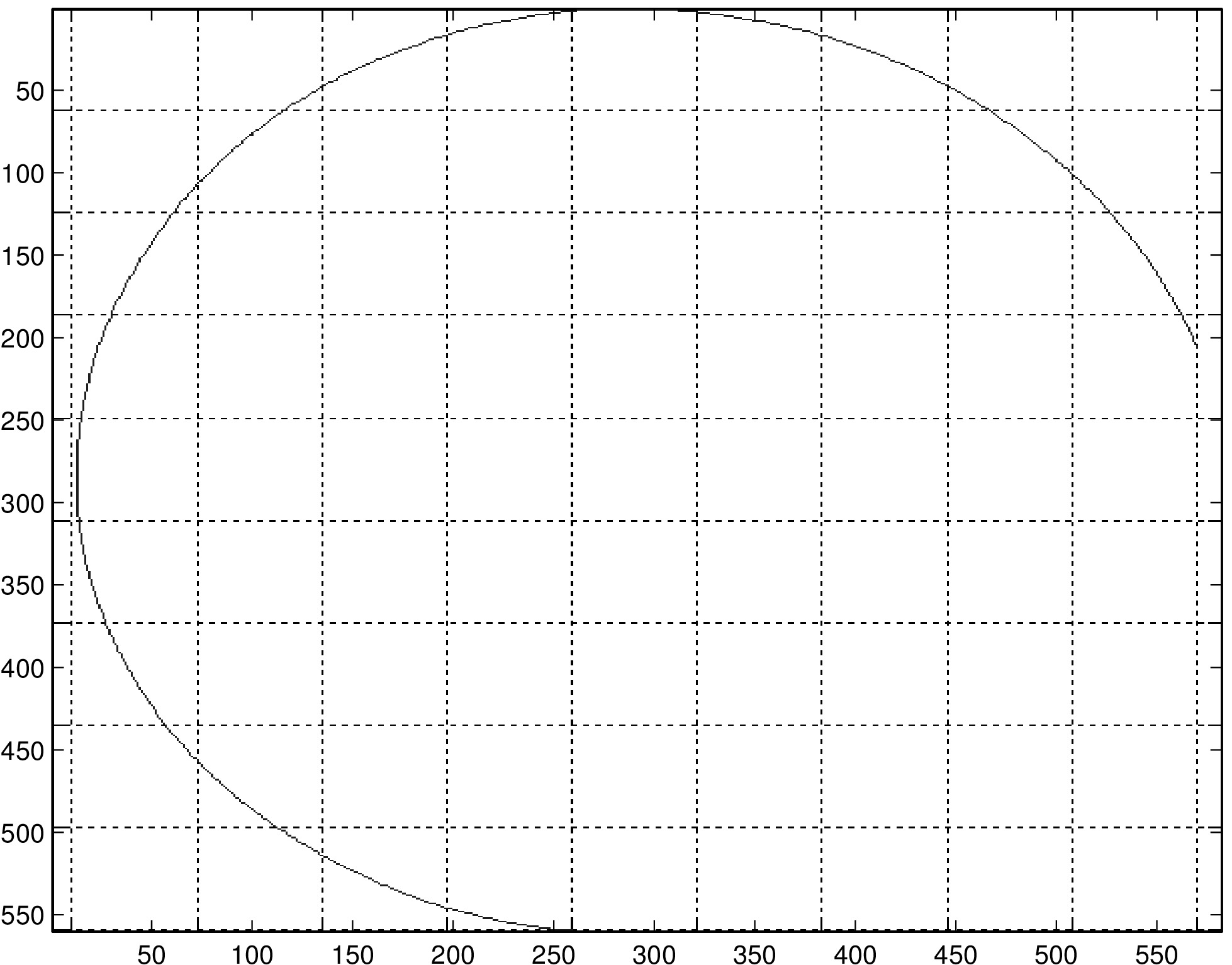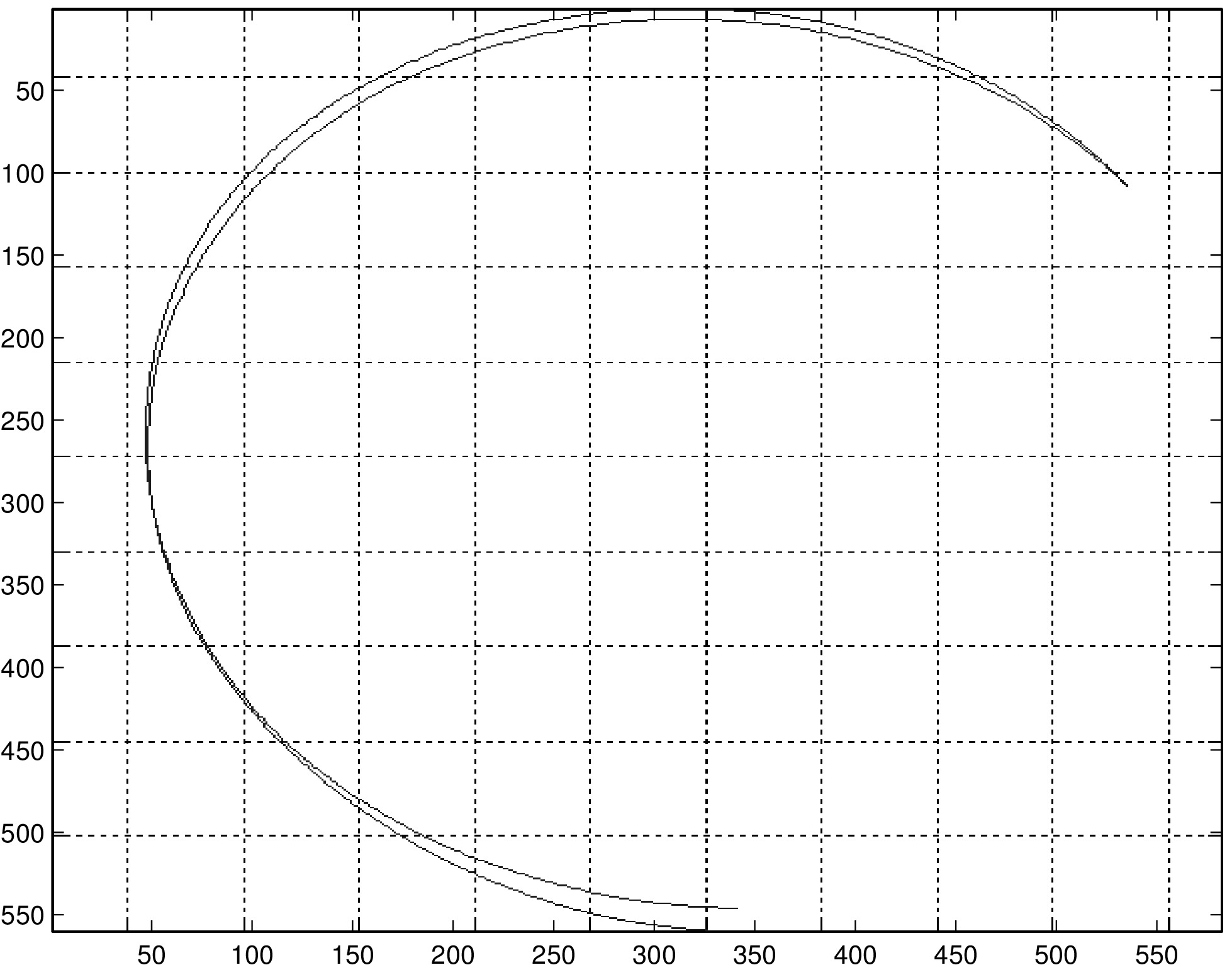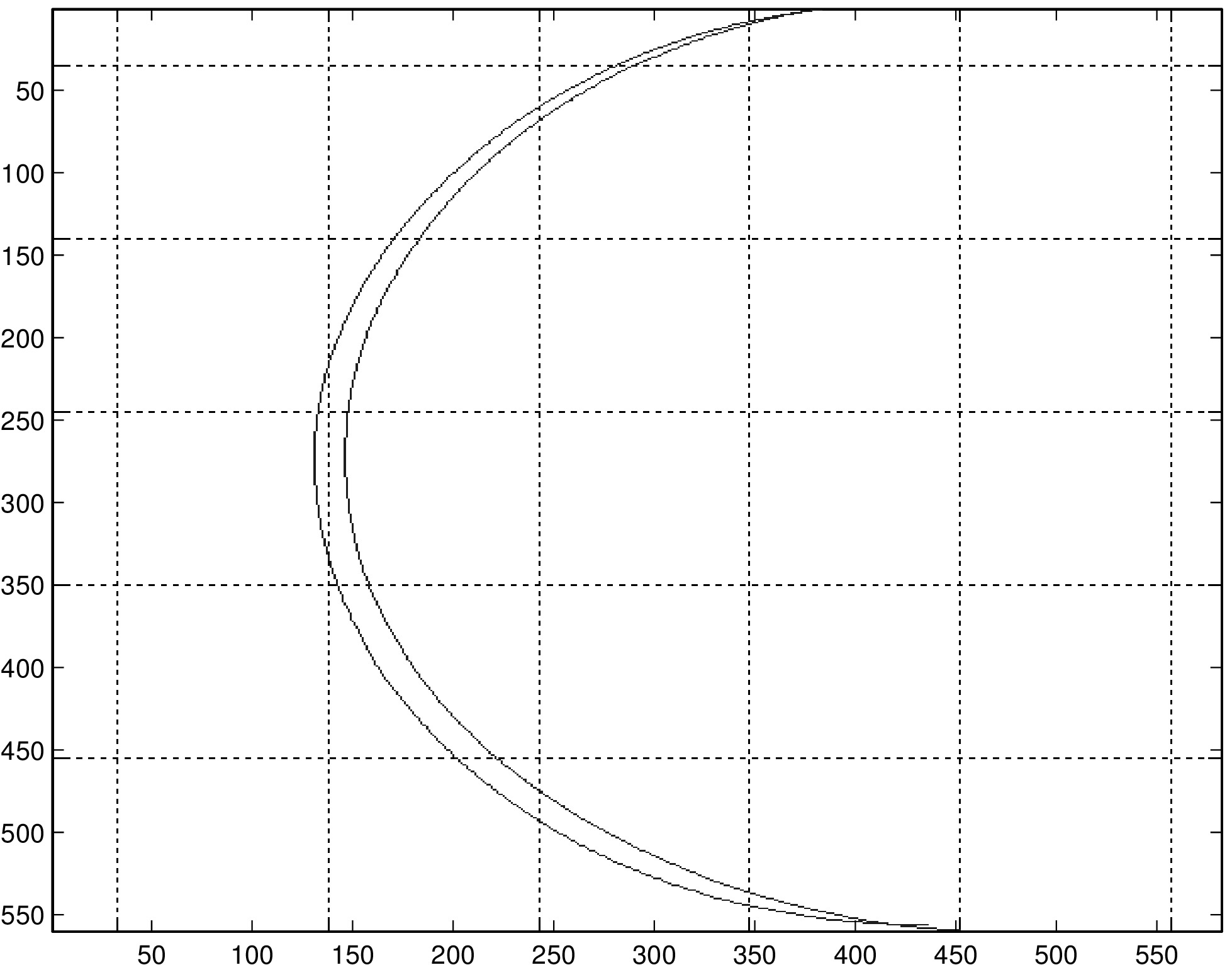Fig 5. Reconstruction of several paths before (left) and after (right) calibration.

## Pairwise PlotsFig 6. Reconstruction of an arc using all pairs of sensors.
##### A major remaining issue is the discrepancy between the path reconstruction obtained by averaging the reconstructions of each pair of sensors, and the reconstructions of the individual sensors. Averaging all sensor pairs, while not yet perfectly accurate, generally yields smooth paths that resemble the true path. Examining the pairwise plots (Figure 7), where the ijth plot is the path of the puck's center computed using only sensors i and j, it is unclear how the accurate path of Figure 6 was reconstructed by averaging the pairwise results. Note that the above figure was drawn without the consistency check, so the apparently unreliable sensors evident in the pairwise plots were not discarded - in fact, when these sensors were discarded (not presented here), the reconstructed path became less accurate. Understanding why some pairwise path reconstructions are so inaccurate, and how the average of all sensors can be so accurate despite unreliable pairwise reconstructions may gave insight into the behavior of the algorithm and the puck.Fig 7. Reconstructions of the arc in Figure 6 using individual pairs of sensors. Note that the path in Figure 6 was obtained by averaging information from each of these pairwise reconstructions.